Search by Topic

Filter by: Content type:
Age range:
Challenge level:

There are 27 results

Broad Topics > Measuring and calculating with units > Area - circles, sectors and segmentsCurvy Areas

Age 14 to 16 Challenge Level:

Have a go at creating these images based on circles. What do you notice about the areas of the different sections?Partly Circles

Age 14 to 16 Challenge Level:

What is the same and what is different about these circle questions? What connections can you make?Gutter

Age 14 to 16 Challenge Level:

Manufacturers need to minimise the amount of material used to make their product. What is the best cross-section for a gutter?Spokes

Age 16 to 18 Challenge Level:

Draw three equal line segments in a unit circle to divide the circle into four parts of equal area.Salinon

Age 14 to 16 Challenge Level:

This shape comprises four semi-circles. What is the relationship between the area of the shaded region and the area of the circle on AB as diameter?An Unusual Shape

Age 11 to 14 Challenge Level:

Can you maximise the area available to a grazing goat?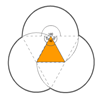Triangles and Petals

Age 14 to 16 Challenge Level:

An equilateral triangle rotates around regular polygons and produces an outline like a flower. What are the perimeters of the different flowers?Approximating Pi

Age 14 to 18 Challenge Level:

By inscribing a circle in a square and then a square in a circle find an approximation to pi. By using a hexagon, can you improve on the approximation?Blue and White

Age 11 to 14 Challenge Level:

Identical squares of side one unit contain some circles shaded blue. In which of the four examples is the shaded area greatest?Compare Areas

Age 14 to 16 Challenge Level:

Which has the greatest area, a circle or a square inscribed in an isosceles, right angle triangle?Age 14 to 16 Challenge Level:

Given a square ABCD of sides 10 cm, and using the corners as centres, construct four quadrants with radius 10 cm each inside the square. The four arcs intersect at P, Q, R and S. Find the. . . .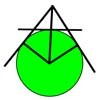Mean Geometrically

Age 16 to 18 Challenge Level:

A and B are two points on a circle centre O. Tangents at A and B cut at C. CO cuts the circle at D. What is the relationship between areas of ADBO, ABO and ACBO?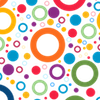Circular Area

Age 7 to 11 Challenge Level:

How could you find out the area of a circle? Take a look at these ways.Efficient Packing

Age 14 to 16 Challenge Level:

How efficiently can you pack together disks?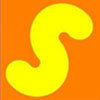Two Shapes & Printer Ink

Age 14 to 16 Challenge Level:

If I print this page which shape will require the more yellow ink?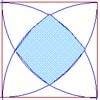Bound to Be

Age 14 to 16 Challenge Level:

Four quadrants are drawn centred at the vertices of a square . Find the area of the central region bounded by the four arcs.Squaring the Circle

Age 11 to 14 Challenge Level:

Bluey-green, white and transparent squares with a few odd bits of shapes around the perimeter. But, how many squares are there of each type in the complete circle? Study the picture and make. . . .Crescents and Triangles

Age 14 to 16 Challenge Level:

Can you find a relationship between the area of the crescents and the area of the triangle?Bull's Eye

Age 11 to 14 Challenge Level:

What fractions of the largest circle are the two shaded regions?F'arc'tion

Age 14 to 16 Short Challenge Level:

At the corner of the cube circular arcs are drawn and the area enclosed shaded. What fraction of the surface area of the cube is shaded? Try working out the answer without recourse to pencil and. . . .The Pillar of Chios

Age 14 to 16 Challenge Level:

Semicircles are drawn on the sides of a rectangle. Prove that the sum of the areas of the four crescents is equal to the area of the rectangle.Floored

Age 11 to 14 Challenge Level:

A floor is covered by a tessellation of equilateral triangles, each having three equal arcs inside it. What proportion of the area of the tessellation is shaded?Round and Round

Age 14 to 16 Challenge Level:

Prove that the shaded area of the semicircle is equal to the area of the inner circle.Square Pegs

Age 11 to 14 Challenge Level:

Which is a better fit, a square peg in a round hole or a round peg in a square hole?Two Circles

Age 14 to 16 Challenge Level:

Draw two circles, each of radius 1 unit, so that each circle goes through the centre of the other one. What is the area of the overlap?Get Cross

Age 14 to 16 Challenge Level:

A white cross is placed symmetrically in a red disc with the central square of side length sqrt 2 and the arms of the cross of length 1 unit. What is the area of the disc still showing?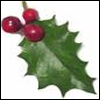Giant Holly Leaf

Age 14 to 16 Challenge Level:

Find the perimeter and area of a holly leaf that will not lie flat (it has negative curvature with 'circles' having circumference greater than 2πr).